## Python: Pig Latin

• If word starts with a vowel, add ‘ay’ to end.
• If word does not start with a vowel, put first letter at the end, then add ‘ay’
• Example:
• word > ordway
• apple > appleay

To further understand and practise the functions in Python, the tutor shared one of the case studies in the online web learning. The case study detail is as above and the workaround is taking the first character and check if it matches the condition.

If yes, then appends the ‘ay’ to the end.
If no, then takes the 2nd character onward, appends the 1st character and follows by appending ‘ay’ to the end.

Sample code as below screenshot: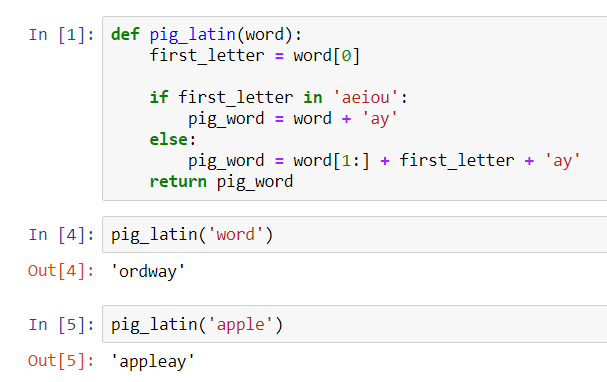## Day 18: Functions in Python

Continuous from the introduction of functions and methods in Python in my previous blog entry. I added a few more examples to understand functions better. In the next examples, I extracted it from DataCamp and Udemy’s online web learning courses.

Built-in Functions
Most of us familiar with len(), str(), format() or print() which I used as examples in previous blog entries. These are built-in Functions from Python which we used to get the length of the string, converting a value to a string and format the value to print out a line accordingly.

Hope, till here, it is simple to go ahead with an example of str() to recap. See below for code snippet and the output from the Jupyter Notebook.

```x = str(10)
print(x)
print(type(x))
```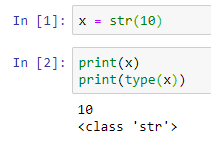It prints out the value 10 as an ‘str’ (string) class. Do you still remember, if we want to print a number, we convert the number to string data type and concatenate with other strings of words (if any) to print out the whole statement? The str() converts number to string. Otherwise, it returns an int data type and it cannot be concatenated with strings.

Now, move on to defining our functions with examples and explanations below. Still remember the keywords for defining a function?

• Keyword def marks the start of function’s header.
• Function name, abc, is uniquely named.
• Parameters (arguments) which will pass values to the function. It is optional. In the example, a and b are the values pass to the function.
Colon (:) marks the end of the function’s header.
• Indentation after the newline.
• Docstring, an optional documentation to describe what the function does, usually helpful to developers.
• Execution statements.
• Return statement to return a value from the function. It is optional.

## Defining a function without a parameter

Let begin to write a simple user defined function with the code snippet below based on the function’s syntax I mentioned above. We choose to leave the parameter as an option, so we do not define it.

```def square():
new_value = 2 ** 2
print(new_value)
print(type(new_value))

square()
```

After writing the function block of codes, we need to call our function. The above function does not do or return anything on the screen unless we do a call or we invoke the function. That is why the next line of code is required:
square()

Upon execution, it prints the value on the screen accordingly. It does not require any input of arguments in this code. And the value is fixated to use,
new_value = 2 ** 2

every time it is being called. it returns the same output.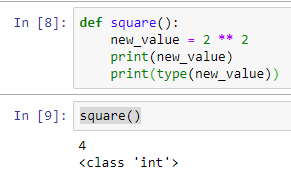## Defining a function with a parameter and provide a default value of a parameter.

How about now, we put in the parameter of our function? How to code it, example below:

```def sayhello(name):
print('hello ' + name)
```

When I call the function sayhello() with an argument, syntax like this,
sayhello(‘Li Yen’)

the output on the screen shows,
hello Li Yen

Up to here, probably, you want to know why sometimes I use parameters and arguments. The DataCamp’s tutorials on Python Data Science Toolbox, gave a good example to example this. The parameter is used when defining a function and we call a function, we pass an argument into the function to be executed. I hope I can make it clear enough and try to distinguish these two jargon.

What if I did not input a value (argument) to the function? The above function expects a value and if we execute it without a value, Python throws an error.

While the parameter defines to accept a value (argument) from the user, it can be set with a default argument by giving it a variable and a value such as name = ‘User’. The variable, name has a value of ‘User’. This is how the code looks like,

```def sayhello(name='User'):
print('hello ' + name)
```

So, when we call the same function again without passing argument, syntax like this,
sayhello()

the output on the screen shows,
hello User

Another example below, showing the combination of using the default value, yet I’m passing my name into the function. It overwrites the value of ‘User’. Cool!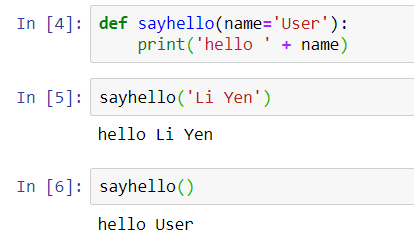## Multiple Function Parameters

Accept more than 1 parameter and when we call a function, number of arguments same as number of parameters. The values are assigned in sequence of the parameters.

Call function: # of arguments = # of parameters. Below is the examples of multiple parameters:

```def raise_to_power(value1, value2):
"""Raise value1 to the power of value2."""
new_value = value1 ** value2
return new_value

result = raise_to_power(2, 3)
print(result)
```
```def square(value1, value2):
new_value = value1 ** value2
return new_value

square(10,3)
```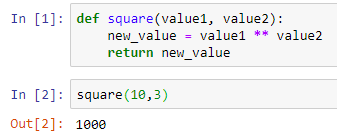Is it straight forward enough with the above example code and output? When the square(10,3) is called, the value of 10 passes to variable, value1 and the value of 3 passes to variable, value2, then the variable new_value holds the value of the computation of value1 ** value2. In syntax format, it looks like this,

```new_value = 10 ** 3
```

To call the square() function, declare a variable result and print the output of result:

```result = square(10,3)
print(result)
```

## Return statement

Return a Boolean from a function
We can return a Boolean from a function, just like other data types too. The example below, I’m returning a Boolean’s value.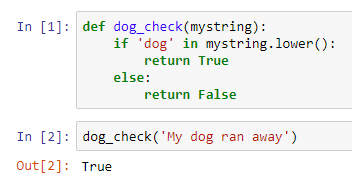If we check the condition as below,

```'dog' in 'My dog ran away'
```

It returns True.

So, in that example, the tutor said, we can simplify the codes by removing the if statement to achieve the same result. It can be done in one line.

```def dog_check(mystring):
return 'dog' in mystring.lower()
```

## Return multiple values

As mentioned in my summary post, the return statement can return more than one value. When I learned it through DataCamp, this can be happened when a function returns a tuple. We covered what tuple is. It is a collection of data, just like a list of parentheses. It is immutable, which means data cannot be changed.
Tuple (‘a’, ‘b’, ‘c’, ‘d’)

Below is the screenshot of the sample code: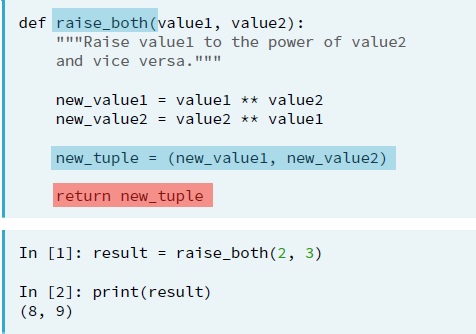Another sample code from DataCamp, an expand from the above square() function to return 2 values in a tuple, unpack the tuple and print out a value using index.

```def square(value1, value2):
new_value1 = value1 ** value2
new_value2 = value2 ** value1

new_tuple = (new_value1, new_value2)
return new_tuple

result = square(10,3)
print(result)
print(result) #print(square(10,3))
print(result) #print(square(10,3))
```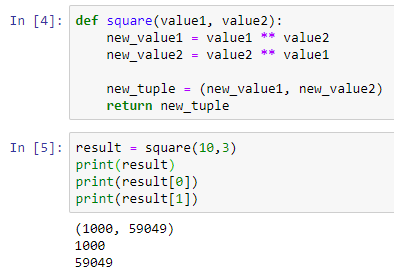The above shows a combination of using functions with multiple parameters, returning the multiple values using a tuple and print out the result by unpacking the tuple and print out the result of new_value1 and new_value2 by its index.

Summary of the day:

• Define function without parameter.
• Define a function with a parameter.
• Use the default argument in the parameter.
• Use the return statement with a single value.
• Use the return statement with tuple for multiple values.
• Unpack tuple and using index slicing to print the value of a tuple.

## Day 16: Python Control Flow using If, Elif and Else StatementsWhen we want to execute the codes with matching certain conditions, If, Elif and Else is being used. It is simple and easy to code. Let me show the syntax. The main keywords are if, elif and else as the logic flow. It uses along with colon (:) and indentation (whitespace). Following is the syntax for if statement, a single condition to be matched:

```if condition :
expression
```

When we have more than one condition to handle, if-else or if-elif-else statement is used. The syntax is as below:

```if condition :
expression
else :
expression
```

if-else statement may look similar to if-elif-else statement, however, the difference is if-else statement check at most two conditions. If anything does not match first (if) statement, then it goes to second (else) statement to process. This is difference compared with if-elif-else statement where it has few if conditions to check and see if any matches. Python starts at the top of the code and run the first block, and that returns a True, Python will run only the first one and exit from the if statement, even there is multiple elif blocks are True.

```if condition :
expression
elif condition :
expression
else :
expression
```

Examples of how to use those syntax:

```# Syntax for if statement
if x = 1:
print('X has a value.')

# Syntax for if/else statement
if x = 1:
print('X has a value.')
else:
print('X does not have a value.')

# Syntax for multiple conditions
if x = 1:
print('X has a value.')
elif x = a:
print('X has a string.')
else:﻿
print('X does not have a value.')
```

The if, elif and else keywords have to be aligned in the same indentation in order to work properly. We can have multiple elif statements for different conditions to be matched. After processing the expression (in this case is the print() statement), the if statement exit the control flow and returns result.

It is a straightforward control flow statement and it is often used with other control flows. Check it out in my next posts.

Summary of the day:

• Control flow: If, Elif and Else